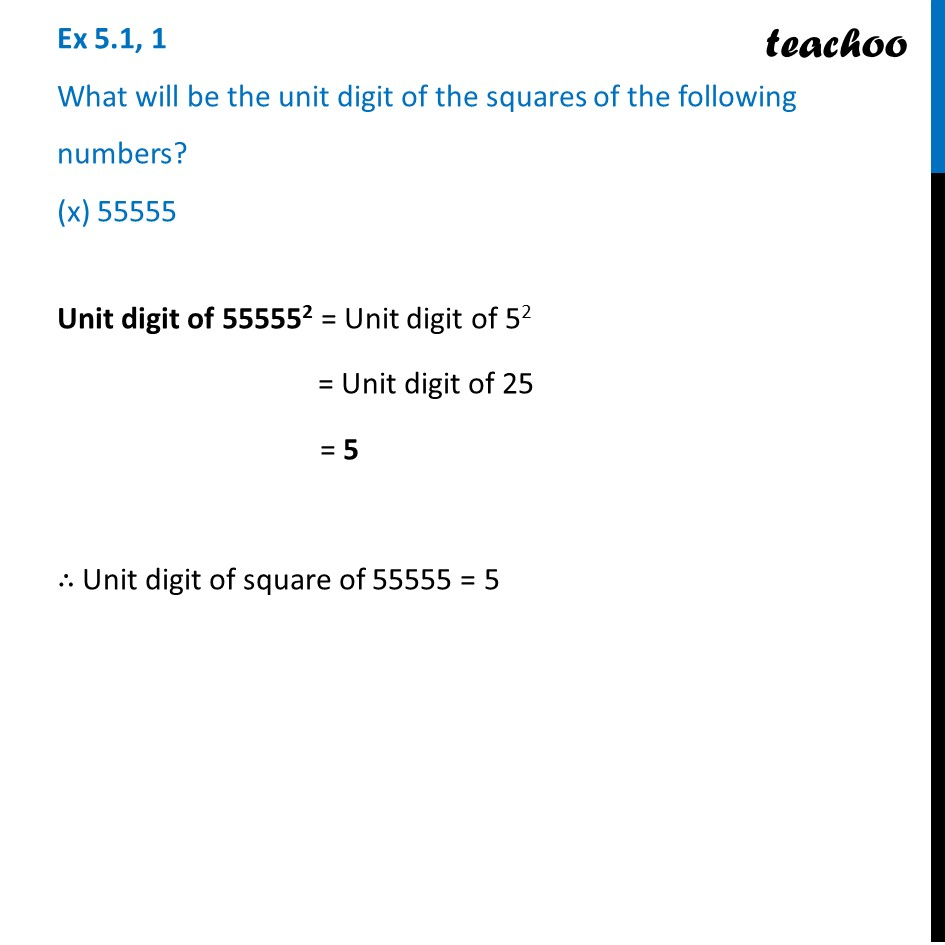Ex 5.1

Chapter 5 Class 8 Squares and Square Roots
Serial order wiseLearn in your speed, with individual attention - Teachoo Maths 1-on-1 Class

### Transcript

Ex 5.1, 1 What will be the unit digit of the squares of the following numbers? (x) 55555Unit digit of 555552 = Unit digit of 52 = Unit digit of 25 = 5 ∴ Unit digit of square of 55555 = 5